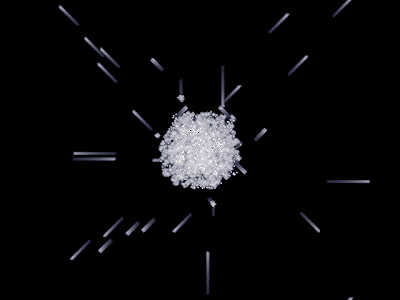### Frequency-based CA visualization (using Single Rotataion as an example)

In the binary cellular automata, cells can have only 2 states: ON and OFF. This, monochrome images are the most natural choice to visualize such automata. While informative, this visualization method produces somewhat dull images, especially when spaceships don't have easily recognizable form. My first idea to make it a bit fancier was to add some motion blur, emphasizing spaceships, and it worked well:Single Rotation rule, with motion blur

Motion blur is effectively a low-pass filter applied to the original BW image. The next idea was to use a combination of several filters to make image more colorful. In my final application, 3 filters were used:

• Low-pass filter, detecting cells that are constantly ON. This filter was assigned to the red channel.
• High-pass filter, detecting cells that are flashing with period 2 (ON-OFF-...). Green channel was used for this filter.
• Band filter (2-nd order), detecting cells, flashing with period 4. If was used for blue.
Together, these 3 filters produce images like this:Single Rotation rule, with 3 different frequency filters for each color channel.

The picture above shows the Single Rotation rule, where random reactions produce many different kinds of spaceships. Interestingly, this colorization scheme allows to distinguish different spaceships by their colors. Finally, by applying these filters to the animation, you'll get the video above. In this video, the field is initialized with random block at the center (at first it appears red because of high cell concentration), and a vertical bar of 2 cells. In the "Single Rotation" rule, such bar of cells is an example of indestructible still life. After the simulation is started, dense random block starts dissipating, emitting multiple spaceships of different kinds. Single Rotation rule supports a lot of them!

For those who prefer higher resolutions, a HD version is available. Full screen and "HD" quality are recommended.

This colorization algorithm can be applied to any other cellular automata too. For example, here is Conway's Life. Note how still lifes are colored in red, and p2 oscillators are green:

## Software

All sources for this post are published at Github:

To use them, clone the repository above, build C++ modules, executing
$make and then run $ python make_color_animation.py
This will create a sequence of animation frames, that can later be converted to video, using ffmpeg. I used the following command line for conversion (not sure it is the best one though):
ffmpeg -i frame%04d.png -movflags faststart -c:v\
libx264 -preset veryfast -bf 2 -g 15 -crf 23\
-pix_fmt yuv420p -r 30 animation.mp4

At the time of writing, settings for the script above are done inside the source code.

## Algorithm

Three discrete time filters are used to detect flashing frequencies:
$$\begin{eqnarray} x_{t+1} &=& k x_t + s_t,\\ y_{t+1} &=& - k y_t + s_t,\\ z_{t+1} &=& i z_t + s_t. \end{eqnarray}$$
Where $$k \approx 0.99$$ is a decay coefficient, $$s_t$$ is a t-th state of the cell (0 or 1), i is a complex unity. Then, RGB color for the pixel is obtained by formulas:
$$\left( \begin{array}{c}r\\g\\b \end{array} \right) = k_\gamma rgb',$$ $$rgb' = \mathbf{K} \left( \begin{array}{c}x \\ |y| \\ |z| \\ \end{array} \right),$$
where K is color transformation matrix and $$k_\gamma$$ is gamma correction coefficient. The latter is calculated like this:
$$k_\gamma = \max(r', g', b')^{\gamma-1}.$$
where $$\gamma$$ is the gamma correction value (in the video above, 0.2 was used). For the top picture, the following colorization matrix was used:
$$\mathbf{K}=\left(\begin{array} 18.7& 0 & 0 \\ 0 & 94 & -15.7 \\ 0 & 0 & 68 \end{array}\right).$$